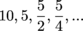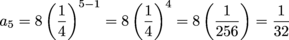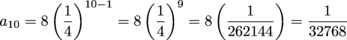## Geometric Sequences

It's our experience that people tend to wig out by the time they get to geometric sequences. Shmoop to the rescue. What else is new? All you need to remember is that while arithmetic sequences add some number to get the next term, geometric sequences multiply each term by some number.

Here's a geometric sequence:

4, 8, 16, 32, 64, …

We're multiplying by 2 each time:

4 × 2 = 8
8 × 2 = 16
16 × 2 = 32
32 × 2 = 64

The constant that we're multiplying each term by (2 in this case) is called the common ratio, which we usually represent with the letter r. Here's another geometric sequence:The common ratio in that geometric sequence is r =. Your turn.

### Sample Problem

Find the common ratio for the sequenceRemember, the common ratio is just the number we multiply by to get to the next term in a geometric sequence. Here, our terms are getting smaller. Instead of multiplying by a whole number, we're going to have to multiply by a fraction. This is a completely normal thing for geometric sequences, by the way. In this sequence, each number is half the previous number, so it's fairly easy to see that we're multiplying by ½.

The other thing it might be helpful to know at this point is the general form of a geometric sequence's explicit rule. It looks like this:

{an} = a1(r)n – 1

This means that the nth term, an, is just the first term multiplied by the common ratio, r, to the (n – 1)th power. You're probably going to want to commit that general form equation thingy to memory. It'll be worth it.

And yeah, geometric sequences can also be written using a recursive rule. Here's the general form:

{an} = (an – 1)(r)

Just multiply the previous term by the common ratio.

### Sample Problem

List the first four terms and the 20th term of a geometric sequence with a first term of 1 and a common ratio of 2.

Let's first find our explicit rule for this thing and then use it to get our terms. Since a1 = 1 and r = 2, we can substitute to get…

an = a1(r)n – 1

an = 1(2)n – 1

Now we can find our first four terms and even our 20th term pretty easily.

a1= 1(2)1 – 1 = 1(2)0 = 1(1) = 1
a
2= 1(2)2 – 1 = 1(2)1 = 1(2) = 2
a
3= 1(2)3 – 1 = 1(2)2 = 1(4) = 4
a4= 1(2)4 – 1 = 1(2)3 = 1(8) = 8…

a20= 1(2)20 – 1 = 1(2)19 = 524,288

So our sequence looks like 1, 2, 4, 8,…, 524,288 ,…

S, Double U, Double E, T.

Oh, and just one thing worth noting in that example. Notice how the first term ended up with a common ratio to the power of zero. This is exactly what allows our general form to work. Since any number to the 0 is just 1, it means our first term will always be a1 times 1, or just a1—every single time.

### Sample Problem

Use the geometric sequence given byto find the 5th and 10th terms.

Oh no. Not the 10th term…You can just go ahead and use the formula on this one.Wow, that's really small. But that also should make sense since the bigger n gets, the smaller the corresponding term will be. We say that the term approaches zero as n approaches infinity. Whoa—calculus.

### Sample Problem

Find the explicit rule for the geometric sequence with a1 = 6 and a4 = ¾.

Finally, a problem we can sink our Shmathematical teeth into. Let's start with what we know. Since a1 = 6, we know that the general form needs to look like…

an = 6(r)n – 1

We also know that a4 = ¾. By substituting 4 for n and ¾ for a4, we can solve for r.Boom. There's our common ratio. Now we can finish our rule:

an = 6(½)n – 1